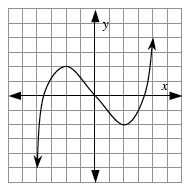### Home > PC > Chapter 7 > Lesson 7.1.1 > Problem7-10

7-10.

Sketch the graph of a function (you do not need to find an equation for it) that is increasing on $\left(−∞, −2\right)$ and $\left(2, ∞\right)$, decreasing on $\left(−2, 2\right)$, concave down on $\left(−∞, 0\right)$, and concave up on $\left(0, ∞\right)$.